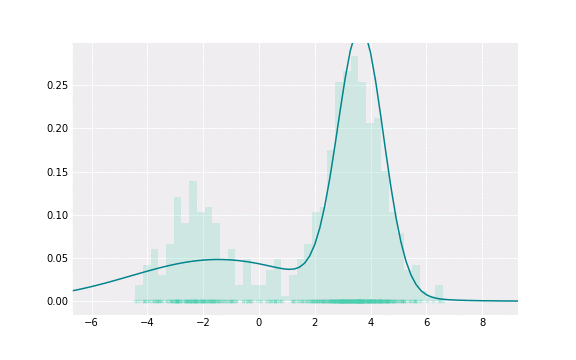# Gaussian Mixture Models Visually ExplainedDensity estimation with Gaussian Mixture. Image by author

A normal distribution is widely known and used in statistics. Because of the central limit theorem the normal distribution explains the data well in many cases. However, in practice the data distribution might be multimodal, i.e. the probability density function has several ‘bumps’. For example, the distribution of hardcover and paperback books prices, as producing a hardcover book is more expensive.

One way to exploit the nice properties of normal distributions for such multimodal data is to use the Gaussian Mixture Model. This combines several normal distributions with different parameters.

The Gaussian Mixture Model is a generative model that assumes…## Anastasia Kireeva

Data Science student at TU Munich